# Colleges with the highest SAT scores in Louisiana

Top 10 colleges in Louisiana with the highest SAT scores
Looking for the colleges with the highest SAT scores in Louisiana? Well you're in luck! We've compiled a national college database and have created a list of the top 10 universities with the highest SAT scores in Louisiana. These are the schools whose applicants had the highest average SAT scores in Louisiana. And, since these tests are meant to determine academic prowess, they are arguably the schools with the most academically proficient students. You could even say these are the best colleges in Louisiana. We also include each college's ACT scores and acceptance rate so that you can see where you would have the easiest or hardest time getting in. Read on to find out more.

## Tulane University SAT scores

The average SAT score for Tulane University is 1420.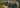The average SAT score of 1420 breaks down into:

• SAT math: 720

The average ACT score for Tulane University is 32 and their acceptance rate is 17.3%.

## Louisiana State University SAT scores

The average SAT score for Louisiana State University is 1180.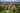The average SAT score of 1180 breaks down into:

• SAT math: 590

The average ACT score for Louisiana State University is 26 and their acceptance rate is 74.2%.

## Louisiana Tech University SAT scores

The average SAT score for Louisiana Tech University is 1160.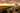The average SAT score of 1160 breaks down into:

• SAT math: 580

The average ACT score for Louisiana Tech University is 24 and their acceptance rate is 61.7%.

## Loyola University New Orleans SAT scores

The average SAT score for Loyola University New Orleans is 1157.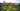The average SAT score of 1157 breaks down into:

• SAT math: 560

The average ACT score for Loyola University New Orleans is 25 and their acceptance rate is 94.3%.

## Centenary College of Louisiana SAT scores

The average SAT score for Centenary College of Louisiana is 1140.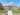The average SAT score of 1140 breaks down into:

• SAT math: 570

The average ACT score for Centenary College of Louisiana is 25 and their acceptance rate is 59.7%.

## University of Louisiana Lafayette SAT scores

The average SAT score for University of Louisiana Lafayette is 1140.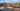The average SAT score of 1140 breaks down into:

• SAT math: 560

The average ACT score for University of Louisiana Lafayette is 23 and their acceptance rate is 55.3%.

## Southeastern Louisiana University SAT scores

The average SAT score for Southeastern Louisiana University is 1140.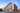The average SAT score of 1140 breaks down into:

• SAT math: 570

The average ACT score for Southeastern Louisiana University is 22 and their acceptance rate is 90.1%.

## University of New Orleans SAT scores

The average SAT score for University of New Orleans is 1140.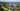The average SAT score of 1140 breaks down into:

• SAT math: 560

The average ACT score for University of New Orleans is 23 and their acceptance rate is 56.6%.

## Nicholls State University SAT scores

The average SAT score for Nicholls State University is 1120.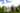The average SAT score of 1120 breaks down into:

• SAT math: 580

The average ACT score for Nicholls State University is 22 and their acceptance rate is 88.4%.

## Xavier University of Louisiana SAT scores

The average SAT score for Xavier University of Louisiana is 1070.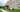The average SAT score of 1070 breaks down into:

• SAT math: 530